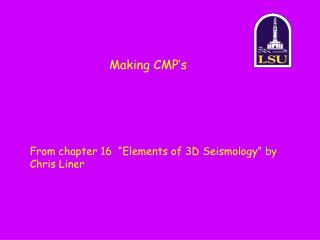DownloadDownload PresentationMaking CMP’s

Making CMP’s

Download PresentationMaking CMP’s

- - - - - - - - - - - - - - - - - - - - - - - - - - - E N D - - - - - - - - - - - - - - - - - - - - - - - - - - -
Presentation Transcript

1. Making CMP’s From chapter 16 “Elements of 3D Seismology” by Chris Liner

2. Outline • Normal Moveout • Stacking

3. Normal Moveout Hyperbola: x T

4. Normal Moveout x T “Overcorrected” Normal Moveout is too large Chosenvelocity for NMO is too (a) large (b) small

5. Normal Moveout x T “Overcorrected” Normal Moveout is too large Chosenvelocity for NMO is too (a)large (b) small

6. Normal Moveout x T “Under corrected” Normal Moveout is too small Chosenvelocity for NMO is (a) too large (b) too small

7. Normal Moveout x T “Under corrected” Normal Moveout is too small Chosenvelocity for NMO is (a) too large (b) too small

8. Vinterval from Vrms Dix, 1955

9. Vrms V1 V2 Vrms < Vinterval V3

10. Vinterval from Vrms

11. Multiples and Primaries x M1 T M2

12. Conventional NMO before stacking x M1 NMO correction V=V(depth) e.g., V=mz + B T M2 “Properly corrected” Normal Moveout is just right Chosenvelocity for NMO is correct

13. Over-correction (e.g. 80% Vnmo) x x M1 M1 NMO correction V=V(depth) e.g., V=0.8(mz + B) T T M2 M2

14. f-k filtering before stacking (Ryu) x x M1 NMO correction V=V(depth) e.g., V=0.8(mz + B) T T M2 M2

15. Correct back to 100% NMO x x M1 M1 NMO correction V=V(depth) e.g., V=(mz + B) T T M2 M2

16. Outline • Convolution and Deconvolution • Normal Moveout • Stacking

17. NMO stretching T0 V1 V2 “NMO Stretching”

18. NMO stretching V1 T0 V2 “NMO Stretching” V1<V2

19. NMO stretching V1 V1<V2 NMO “stretch” = “linear strain” V2 Linear strain (%) = final length-original length original length X 100 (%)

20. NMO stretching original length = final length = V1 V1<V2 V2 X 100 (%) NMO “stretch” = X 100 (%)

21. stretching for T=2s,V1=V2=1500 m/s Green line assumes V1=V2 Blue line is for general case, where V1, V2 can be different and delT0=0.1s (this case: V1=V2) Matlab code X 100 (%)

22. Stacking + + =

23. Semblance Analysis X + + = Twtt (s) “Semblance”

24. Semblance Analysis X V + + = V1 V2 Twtt (s) V3 Peak energy